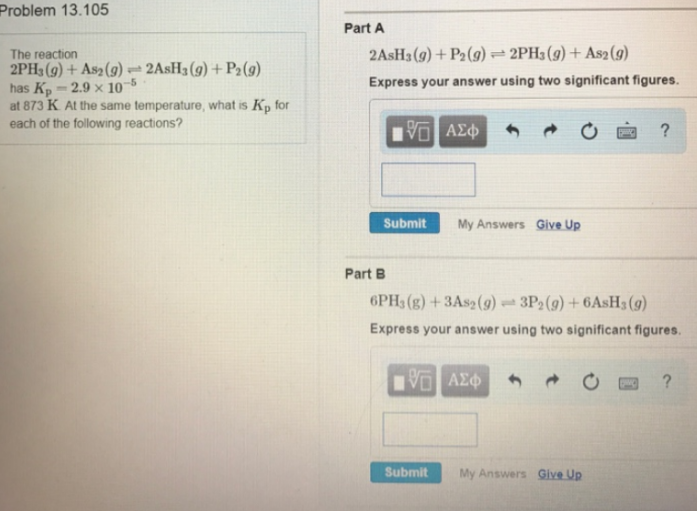# Problem: The reaction 2PH3 (g) + As2 (g) ⇌ 2AsH3 (g) + P2 (g) has Kp = 2.9 x 10-5 at 873 K. At the same temperature, what is Kp for each of the following reactions? 2AsH3 (g) + P2(g) ⇌ 2PH3 (g) + As2(g) Express your answer using two significant figures. 6PH3(g) + 3As2(g) ⇌ 3P2(g) + 6AsH3(g) Express your answer using two significant figures.

###### FREE Expert Solution
88% (423 ratings)###### Problem Details

The reaction

2PH3 (g) + As2 (g) ⇌ 2AsH3 (g) + P2 (g)

has Kp = 2.9 x 10-5 at 873 K. At the same temperature, what is Kp for each of the following reactions?

2AsH3 (g) + P2(g) ⇌ 2PH3 (g) + As2(g)

6PH3(g) + 3As2(g) ⇌ 3P2(g) + 6AsH3(g)